# IB DP Physics: HL复习笔记7.1.4 Isotopes & Radioactive Decay

### Isotopes

#### AZX Notation

• A nuclide is a group of atoms containing the same number of protons and neutrons
• For example, 5 atoms of oxygen are all the same nuclide but are 5 separate atoms
• Atomic symbols are written in a specific notation called nuclide or AZX notation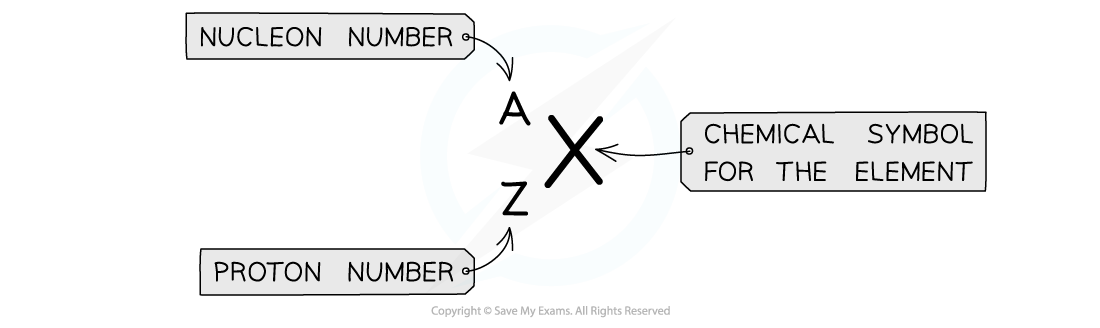Atomic symbols in AZX Notation describe the constituents of nuclei

• The top number A represents the nucleon number or the mass number
• Nucleon number (A) = total number of protons and neutrons in the nucleus
• The lower number Z represents the proton or atomic number
• Proton number (Z) = total number of protons in the nucleus
• Note: In Chemistry, the nucleon number is referred to as the mass number and the proton number as the atomic number. The periodic table is ordered by atomic number

#### Isotopes

• Although all atoms of the same element always have the same number of protons (and hence electrons), the number of neutrons can vary
• An isotope is defined as:

An atom (of the same element) that has an equal number of protons but a different number of neutrons

• Hydrogen has two isotopes: deuterium and tritium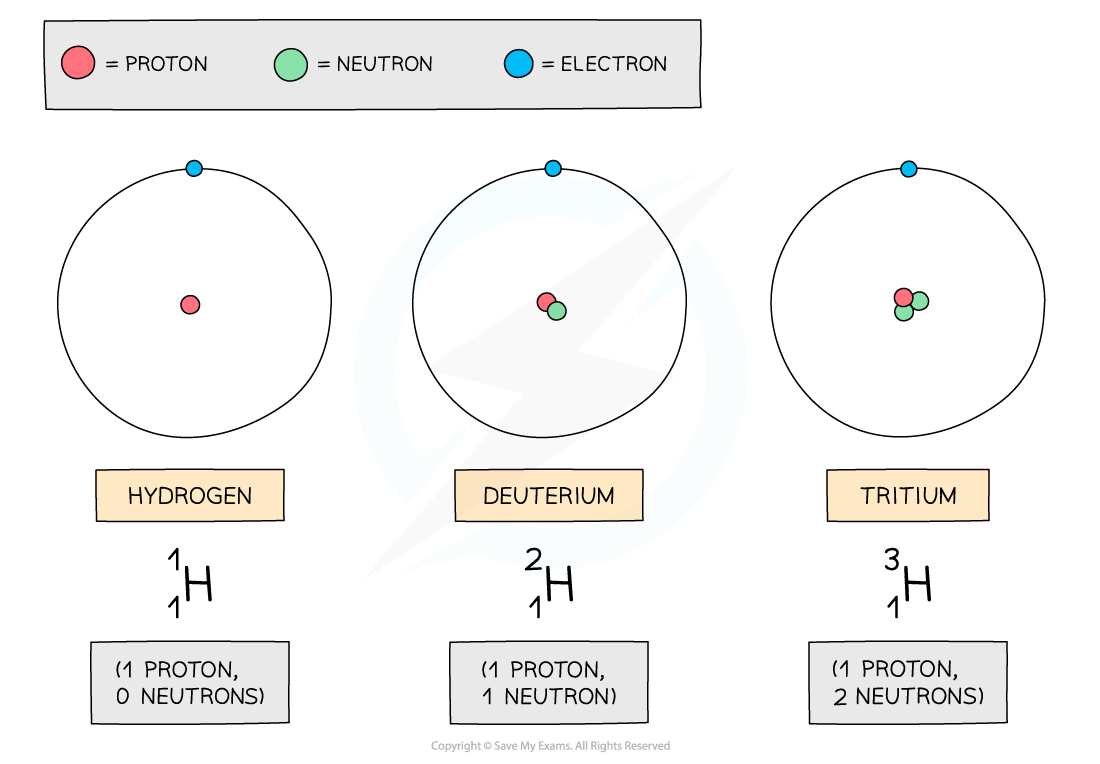The three atoms shown above are all forms of hydrogen, but they each have different numbers of neutrons

• The neutron number of an atom is found by subtracting the proton number from the nucleon number
• Since nucleon number includes the number of neutrons, an isotope of an element will also have a different nucleon / mass number
• Since isotopes have an imbalance of neutrons and protons, they are unstable
• This means they constantly decay and emit radiation to achieve a more stable form
• This can happen from anywhere between a few nanoseconds to 100,000 years

#### Differences Between Isotopes

• The number of neutrons in an atom does not affect the chemical properties of an atom, such as its charge, but only its mass
• This is because neutrons have no charge but do have mass
• The charge of the nucleus of a particular element is always the same
• In the periodic table, the mass number of Chlorine is often given as 35.5This section of a periodic table shows Chlorine as having a mass number of 35.5, but other elements have an integer mass number

• The mass number of Chlorine is given as 35.5 because it has roughly equal numbers of isotopes with a mass number of 35, and of 36
• The number of electrons and protons in different isotopes remains the same
• Isotopes tend to be more unstable due to the imbalance of protons and neutrons

#### Isotopic Data

• Isotopic data is defined as:

The relative amounts of different isotopes of an element found within a substance

• It is used to identify an isotopic signature within organic and inorganic materials
• Isotopic data is often used for determining the age of archaeological findings and is used in radioactive dating
• Carbon–14 is a naturally occurring isotope most often used for this, since it is present in all living beings and undergoes radioactive decay
• When a plant or animal dies, the natural decay of this isotope means the concentration of the carbon–14 in its tissue gradually reduces
• Since carbon–14 has a long half-life of around 6000 years, the half-life can be used to determine the age of the plant or animal when it died

#### Worked Example

One of the rows in the table shows a pair of nuclei that are isotopes of one another.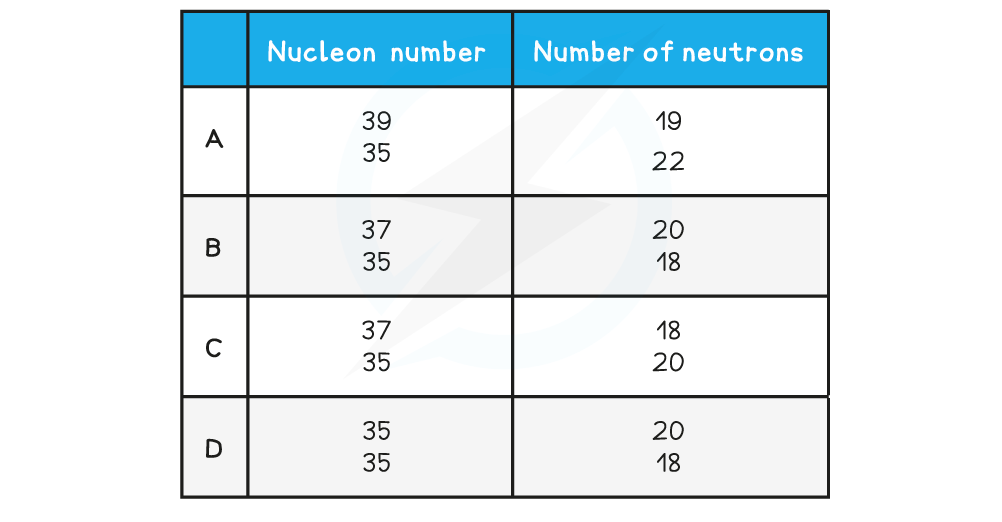Which row is correct?

Step 1: Properties of isotopes

Isotopes are nuclei with the same number of protons but different number of neutrons

The nucleon number is the sum of the protons and neutron

Therefore, an isotope has a different nucleon number too

Step 2: Calculate protons in the  first nucleus

Nucleon number: 37

Neutrons: 20

Protons = 37 − 20 = 17

Step 3: Calculate protons in the second nucleus

Nucleon number: 35

Neutrons: 18

Protons = 35 − 18 = 17

Step 4: Conclusion

Therefore, they have the same number of protons but different numbers of neutrons and are isotopes of each other

The correct answer is therefore option B

#### The Random Nature of Radioactive Decay

• Radioactive decay is defined as:

The spontaneous disintegration of a nucleus to form a more stable nucleus, resulting in the emission of an alpha, beta or gamma particle

• The random nature of radioactive decay can be demonstrated by observing the count rate of a Geiger-Muller (GM) tube
• When a GM tube is placed near a radioactive source, the counts are found to be irregular and cannot be predicted
• Each count represents a decay of an unstable nucleus
• These fluctuations in count rate on the GM tube provide evidence for the randomness of radioactive decay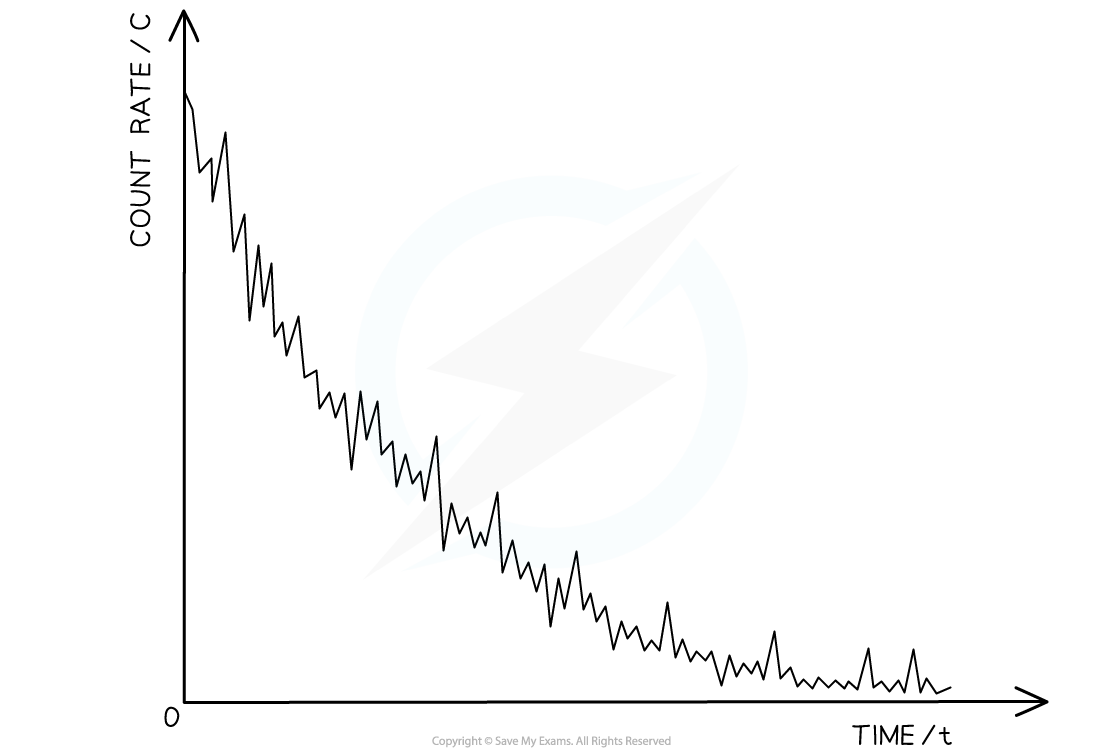The variation of count rate over time of a sample radioactive gas. The fluctuations show the randomness of radioactive decay

• Radioactive decay is both spontaneous and random
• A spontaneous process is defined as:

A process which cannot be influenced by environmental factors

• This means radioactive decay cannot be affected by environmental factors such as:
• Temperature
• Pressure
• Chemical conditions
• A random process is defined as:

A process in which the exact time of decay of a nucleus cannot be predicted

• Instead, the nucleus has a constant probability, ie. the same chance, of decaying in a given time
• Therefore, with large numbers of nuclei, it is possible to statistically predict the behavior of the entire group

#### Nuclear Stability Graph

• The most common elements in the universe all tend to have values of N and Z less than 20 (plus iron which has Z = 26, N = 30)
• Where:
• N = number of neutrons
• Z = number of protons / atomic number
• This is because lighter elements (with fewer protons) tend to be much more stable than heavier ones (with many protons)
• Nuclear stability becomes vastly clearer when viewed on a graph of N against Z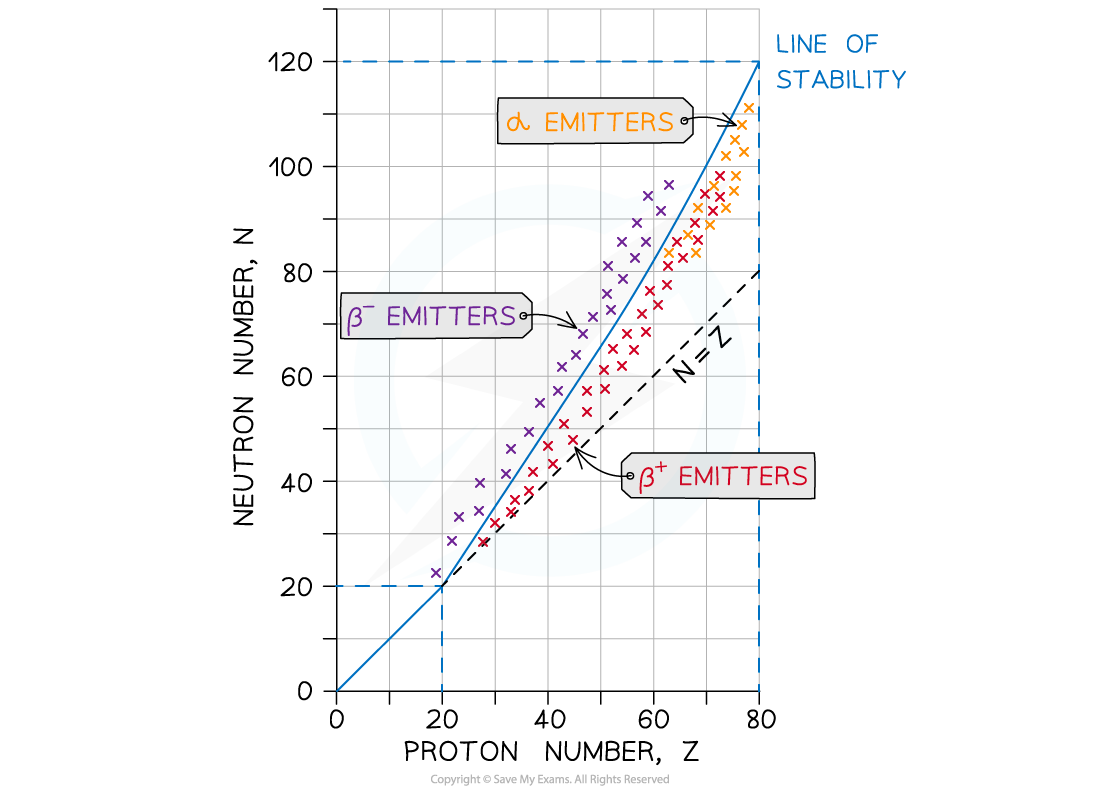This nuclear stability curve shows the line of stable isotopes and which unstable isotopes will emit alpha or beta particles

• A nucleus will be unstable if it has:
• Too many neutrons
• Too many protons
• Too many nucleons ie. too heavy
• Too much energy
• An unstable atom wants to become stable
• For light isotopes, Z < 20:
• All these nuclei tend to be very stable
• They follow the straight-line N = Z
• For heavy isotopes, Z > 20:
• The neutron-proton ratio increases
• Stable nuclei must have more neutrons than protons
• This imbalance in the neutron-proton ratio is very significant to the stability of nuclei
• At a short range (around 1–4 fm), nucleons are bound by the strong nuclear force
• Below 1 fm, the strong nuclear force is repulsive in order to prevent the nucleus from collapsing
• At longer ranges, the electromagnetic force acts between protons, so more protons cause more instability
• Therefore, as more protons are added to the nucleus, more neutrons are needed to add distance between protons to reduce the electrostatic repulsion
• Also, the extra neutrons increase the amount of binding force which helps to bind the nucleons together